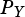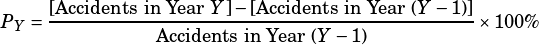The bar graph above shows the number of reported automobile accidents in Milwaukee, Wisconsin from 2010 to 2019. A traffic analyst calculates the percent change for each year,, using the following formula:For example, for she calculates the percent change for 2014 as follows:Which of the following will have the least value?

A)
B)
C)
D)